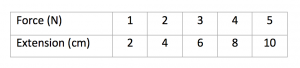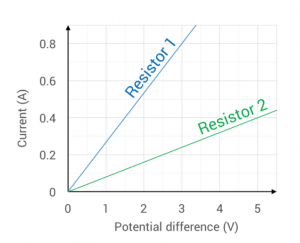Physics 18/01/2019

# Command words in GCSE Physics

By KEVIN QUINN

Command words tell you what you have to do in each question. Spotting these words as soon as you first scan a question – and thinking about what they mean – will both save you time and get you extra marks in the GCSE Physics exam.

## State

A state (or give or name) question is the most straightforward type of command-word question in the exam. To answer it, you simply have to recall a fact, definition or equation and write it down.

EXAMPLE. State the law of reflection .

ANSWER. When a wave strikes a surface, the angle of reflection is equal to the angle of incidence .

Keep your answers concise. In answer to the question above you could add that the angle of reflection is the angle between the normal and the reflected ray, that the angle of incidence is the angle between the incident ray and the normal, and that this normal is an imaginary line at 90° to the surface. This additional information would be correct, but it won’t pick up any extra marks in this 1-mark question!

## Describe

In a describe question, you simply have to say what you see (or what happened or what will happen). You won’t be awarded any marks for attempting to explain anything. Describe questions often come after tables and graphs, as shown in the following example.

EXAMPLE 1. Fiona investigates how the extension of a spring is affected by the force which is applied to it and obtains the following results. Describe how the extension is affected by the force applied .ANSWER. As the force increases, so too does the extension  in direct proportion (or as the force doubles, so too does the extension) .

EXAMPLE 2. Eric has just blown up a balloon. The air inside the balloon is at a temperature of 18 °C. Describe what will happen if he places the balloon into a freezer which is at a temperature of -18 °C .

ANSWER. The volume of the balloon will decrease .

## Explain

Explain questions are usually more difficult (and so are normally awarded more marks) than describe questions. If a describe question asks you what happened, then the matching explain question will ask you why it happened.

EXAMPLE. Explain your answer to the previous question (about what happens when the balloon is placed into the freezer) .

ANSWER. As the temperature of the air inside the balloon decreases, so too does its pressure . Because the pressure inside the balloon is lower than that of the surrounding air , a force will be exerted on the balloon which will cause its volume to decrease . (When the pressures inside and outside the balloon are equal to one another, this resultant force will fall to zero and the volume of the balloon will no longer decrease.)

Watching My GCSE Science videos, doing lots of exam-style questions and reviewing the mark schemes will help you learn how to answer explain questions.

You may be asked to explain something which seems unfamiliar. The key here is to identify the part of the course that the question relates to and then apply your knowledge of physics to the unfamiliar context.

## Calculate

In a calculate question, you will normally have to choose the correct equation to use, substitute the right numbers into the right places, and write down your final answer. You may also be asked to include the correct unit with your answer, or to write it to a certain number of significant figures. For more on units and significant figures, see our blog Dealing with numbers in physics.

EXAMPLE. For every 100 J of energy supplied to an electric toaster, 96 J are transferred to its thermal energy store. Calculate the efficiency of the toaster.

ANSWER. Efficiency = (useful output energy ÷ total input energy) = 96 ÷ 100 = 0.96 (96%).

## Show that

Show that questions are similar to calculate questions but with one key difference. When a show that question appears in your exam, you will often have to use the answer that is provided in a later part of the same question.

In the example below, even if you were unable to show that the final velocity of the car was 36 m/s, you could still have gone on to calculate its kinetic energy (using the equation ½ m v2). What this means is that you should never skip ahead because you can’t answer a single show that question. Always attempt all parts of each question.

EXAMPLE. A racing car of mass 2000 kg accelerates from rest at 1.2 m/s2 for half a minute. Show that it is travelling at a velocity of 36 m/s after this time .

ANSWER. Final velocity = initial velocity + acceleration × time  = 0 + 1.2 × 30 = 36 m/s .

## Evaluate

In an evaluate question, you have to write down all the advantages (pros) and disadvantages (cons) of something. The same number of marks will normally be awarded for each of these.

EXAMPLE. At present, roughly one-fifth of the electricity produced in the UK is generated in nuclear power stations. Evaluate the possibility of increasing the proportion of UK electricity which is generated in this way .

ANSWER. This would allow UK energy demands to be met for many years to come (although uranium is non-renewable, it is quite abundant) . It might reduce reliance on fossil fuels, which would reduce the amounts of greenhouse gases which are released into the atmosphere (and so potentially reduce the effect of human activity of climate change) . However, nuclear power stations are expensive to build (and decommission), the radioactive waste produced is difficult (and expensive) to dispose of, power stations and waste sites could potentially be targeted by terrorists, and there is a small chance that they could undergo meltdown (which could release large amounts of radioactive material into the atmosphere) [any 2].

## Compare

In a compare question, you have to write down the similarities and the differences between two (or more) things. Generally, a mark scheme will award the same number of marks for the similarities and differences, so be sure to include both.

EXAMPLE. Noorah investigates the current-potential difference (I-V) behaviour of two resistors and obtains the results below. Compare the I-V behaviour of both resistors over the range of values shown .ANSWER. The current through each resistor increases as the potential difference across it is increased . However, at a given potential difference, the current through resistor 1 is greater than that through resistor 2 / the resistance of resistor 1 is lower than that of resistor 2 .

## Suggest

In a suggest question, you will have to apply your knowledge and understanding to a new situation. In this example, although the fuel efficiency of a car isn’t mentioned in the specification, you should know that air resistance, friction and unnecessary acceleration and braking will all increase the amount of work which its engine will have to do in getting from A to B.

EXAMPLE. Suggest one way in which the fuel efficiency of a car can be improved .

ANSWER. The body of the car could be streamlined more (so that the air resistance acting on it when it is travelling at a given speed is decreased), it should be driven as steadily as possible (without unnecessary acceleration and braking), it should be serviced regularly (so that there is sufficient lubricating oil in the engine, for example), etc. [any 1].

## Discuss

Discuss questions can be tricky since they are more open-ended than most other types of questions. To answer them successfully, you should have a good knowledge of the question topic and pay close attention to the wording of the question and the number of marks available.

EXAMPLE. Discuss some of the uses of gamma rays and x-rays in the diagnosis (detection) of medical conditions and diseases .

ANSWER. Gamma rays are used in medical tracers; these allow medical staff to investigate processes within the body . These scans rely on the fact that gamma rays (are weakly-penetrating and can) pass easily out of the body . X-rays are used to produce images of suspected broken bones  since they pass easily through soft tissue but are absorbed effectively by bone .

In this example, it’s sensible to assume that there will be two marks available for each of the types of electromagnetic waves mentioned, so you should be sure to include two clear points for each. The question is asking about the uses of gamma rays and x-rays for the diagnosis (detection) of conditions and diseases only, so you won’t be awarded any marks for talking about their uses in treatment (such as in radiotherapy).

Kevin Quinn